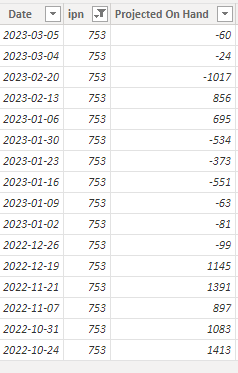cancel
Showing results for
Did you mean:Regular Visitor

## Calculate the count of first consecutive negative values

Hi All,

I'm trying to find the count of the first consecutive negative valuesAs you see in the image I've selected one ipn and we have projected on-hand values by date I need the count from 19 Dec 2022 to 23 Jan 2023 which is 6 and these are the first consecutive negative values for the projected on-hand.
Can anyone help me with this I need a measure/ calculated column to solve it?

1 ACCEPTED SOLUTIONCommunity Support

Hi @Pavanace16 ,

According to your description, here are my steps you can follow as a solution.

(1)This is my test data.(2)We can create  a calculated column.

``````Flag =
MAXX (
FILTER (
'Table',
'Table'[Date] <= EARLIER ('Table'[Date] )
&& 'Table'[Projected On Hand]> 0
),
'Table'[Date]
)``````
``````rows =
IF (
'Table'[Projected On Hand] < 0,
COUNTROWS( (
FILTER (
'Table',
'Table'[Projected On Hand]<0
&& 'Table'[Flag]= EARLIER ( 'Table'[Flag] )
)
)
))``````

(3)We can create  a measure.

``````Measure 2 =

var _a = MINX(FILTER('Table',[Projected On Hand]<0),[Date])

var _b = MINX(FILTER('Table',[Date]>_a&&[Projected On Hand]>=0),[Date])

return CALCULATE(COUNT('Table'[Date]),FILTER('Table',[Date]>=_a&&[Date]<_b))``````

(3) Then the result is as follows.Best Regards,

Neeko Tang

If this post helps, then please consider Accept it as the solution to help the other members find it more quickly.Community Support

Hi @Pavanace16 ,

According to your description, here are my steps you can follow as a solution.

(1)This is my test data.(2)We can create  a calculated column.

``````Flag =
MAXX (
FILTER (
'Table',
'Table'[Date] <= EARLIER ('Table'[Date] )
&& 'Table'[Projected On Hand]> 0
),
'Table'[Date]
)``````
``````rows =
IF (
'Table'[Projected On Hand] < 0,
COUNTROWS( (
FILTER (
'Table',
'Table'[Projected On Hand]<0
&& 'Table'[Flag]= EARLIER ( 'Table'[Flag] )
)
)
))``````

(3)We can create  a measure.

``````Measure 2 =

var _a = MINX(FILTER('Table',[Projected On Hand]<0),[Date])

var _b = MINX(FILTER('Table',[Date]>_a&&[Projected On Hand]>=0),[Date])

return CALCULATE(COUNT('Table'[Date]),FILTER('Table',[Date]>=_a&&[Date]<_b))``````

(3) Then the result is as follows.Best Regards,

Neeko Tang

If this post helps, then please consider Accept it as the solution to help the other members find it more quickly.